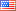# Revision history [back]

### Integral help cos and exp

I'm sure this integral has been seen before. I'm trying to do a convolution for optics homework. No answer returns

sage: var('x1, x2, y1, y2, L, z, r') sage: integral(cos(x1/L)exp(-i(k/z)(x1x2 + y1*y2)), x1, -L/2, L/2)

thanks for any help!2 No.2 RevisionShashank1897 ●30 ●50 ●83

### Integral help cos and exp

I'm sure this integral has been seen before. I'm trying to do a convolution for optics homework. No answer returns

sage: var('x1, x2, y1, y2, L, z, r')
sage: integral(cos(x1/L)exp(-i(k/z)(x1x2 integral(cos(x1/L)*exp(-i*(k/z)*(x1*x2 + y1*y2)), x1, -L/2, L/2)L/2)


thanks for any help!